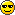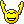# another subnetting question

Member Posts: 30 ■■□□□□□□□□
so i been doing the testout ccna and im alright and i understand most of the stuff. however today i got to the subnetting part and watched the videos, i understood most of it but then when the questions for subnetting came i was baffled because what the instructor had said was the opposite of what the questions were saying. for example if a question asks how many subnets can exist within a given ip address and subnet mask the instructor said use the formula (2^n)-2.
however when the questions came up they were not using (2^n)-2 but rather just 2^n.
however they did use (2^n)-2 on finding out how many hosts could exist.

so im confused here as to why the instructor said use the formula (2^n)-2 for finding how many subnets there could be yet the questions just used 2^n.

thanks

• With "ip subnet zero" configured which is the default, you use 2^n for subnets.

If "no ip subnet zero" is configured, you use 2^n-2, like as the ip hosts.
But nowadays, you use 2^n for subnets and 2^n-2 for the hosts.

Before, in the "old days", the subnet 0 and the last subnet were removed from the subnet ranges and the command "ip subnet zero" appeared to "correct" this and make the subnet 0 and the last subnet of a range available. And it became on by default afterwards
[X] - 350-001 (300h)
[ ] - Lab exam (60h)
Personal blog: http://www.tommyf.net/
• Member Posts: 30 ■■□□□□□□□□
what does " ip subnet zero" mean?
• Member Posts: 20 ■□□□□□□□□□
I've just started my ccna studies, so please correct me if I'm wrong. 2^n-2 will give you the available hosts for the subnet (subtracting the network address and broadcast address).
• Member Posts: 30 ■■□□□□□□□□
cspster wrote: »
I've just started my ccna studies, so please correct me if I'm wrong. 2^n-2 will give you the available hosts for the subnet (subtracting the network address and broadcast address).
thats how i understood it. however the instructor in the video also uses this formula for finding the subnets but in the questions for that section that formula is not used.
• saied45 wrote: »
what does " ip subnet zero" mean?

Here's some great explanations

https://learningnetwork.cisco.com/message/67847
[X] - 350-001 (300h)
[ ] - Lab exam (60h)
Personal blog: http://www.tommyf.net/
• Member Posts: 20 ■□□□□□□□□□
2^n will give you the block size. example: 2^7= 128. divide 256 by the block size and you get your subnets. example: 256/128=2. 2 subnets and 2^7-2= 126 hosts in each subnet.
Take a look at todd lammle's dvd on subnets and vlsm. its pricey, \$150 but well worth it.
• Member Posts: 30 ■■□□□□□□□□
cspster wrote: »
2^n will give you the block size. example: 2^7= 128. divide 256 by the block size and you get your subnets. example: 256/128=2. 2 subnets and 2^7-2= 126 hosts in each subnet.
Take a look at todd lammle's dvd on subnets and vlsm. its pricey, \$150 but well worth it.

are you sure. that doesnt sound right?
• Member Posts: 20 ■□□□□□□□□□
I think so. But like I said I just started my ccna studies too. I hope I'm right.
• Member Posts: 30 ■■□□□□□□□□
for example the question below.
given the network address plus the subnet mask how many subnets can you have and how many hosts per subnet:
172.16.0.0/22

in this question you mask would be 255.255.252.0
you will have 64 subnets and 1022 hosts. here is how you get the results

your ip is a class B so you would get your numbers from the 3rd octate.

you get 22 by adding (+(+(6)+(0)= 255.255.252.0( hit me up if u dont know how to get that).
now since you got 6 on the third octate( number of bits) you use 2^6= 64.
and 64 is the number of subnets. now to figure out the number of hosts, you have 2 remaining from the third octate and 8 remaining from the 4th octate so equals 10
then you will use (2^10)-2= 1024-2=1022
thats what the guy in the video said. explaining it in writing is much harder. but hit me up if u dont understand and i will try to explaine it
• Member Posts: 20 ■□□□□□□□□□
for example the question below.
given the network address plus the subnet mask how many subnets can you have and how many hosts per subnet:
172.16.0.0/22

in this question you mask would be 255.255.252.0

11111111.11111111.11111100.00000000
n= number of 0's left in 3rd octet
2^2=4
block size of 4
256/4= 64
subnets 64

hosts
n= total number of 0's in
2^10 - 2= 1022
1022 hosts

I've made flashcards and started to memorize everything.
• cspster wrote: »
2^n will give you the block size. example: 2^7= 128. divide 256 by the block size and you get your subnets. example: 256/128=2. 2 subnets and 2^7-2= 126 hosts in each subnet.
Take a look at todd lammle's dvd on subnets and vlsm. its pricey, \$150 but well worth it.

There is plenty of information on this site that can/will teach you to subnet without spending a penny.I don't claim to be an expert, but I sure would like to become one someday.

Quest for 11K pages read in 2011
Page Count total to date - 1283
• Member Posts: 30 ■■□□□□□□□□
so i have this problem and i cant figure it out. anyone wants to help me figure this out

• saied45 wrote: »
so i have this problem and i cant figure it out. Anyone wants to help me figure this out

Network : 202.500.55.96
Useable hosts : 202.500.55.97-126
Bcast: 202.200.55.127
[X] - 350-001 (300h)
[ ] - Lab exam (60h)
Personal blog: http://www.tommyf.net/
• Member Posts: 30 ■■□□□□□□□□
• Member Posts: 143 ■■■□□□□□□□
saied45 wrote: »

Network : 202.500.55.96
Useable hosts : 202.500.55.97-126
Bcast: 202.200.55.127

They have given you the subnet. You need to find the increment, so you can find the broadcast address.

You are borrowing three bits in the fourth octet with a 224 subnet
128+64+32= 224 or
128 64 32 16 8 4 2 1
1 1 1 0 0 0 0 0

The last bit is under 32, so your increment is 32.
subnets = 0, 32, 64, 96, 128

You know that the subnet you are working with is 96 and the next subnet is 128.

You add one to 96, which is 97, because you can't give a host a subnet number.

I hope this helps.
• saied45 wrote: »
so i have this problem and i cant figure it out. anyone wants to help me figure this out

find the interesting octect.... the non 255 one
224 is the interesting octect subtract the value from 256 and you get the block size 256-224 = 32

also if you divide 256 by the (block size) 32 you get 8 = the number of subnets available

202.200.55.0
202.200.55.32
202.200.55.64
202.200.55.96
202.200.55.128
202.200.55.160
202.200.555.192
202.200.55.224

In this example the question is answered easily since they give you the subnet.
The first available host is always one more than the subnet
the broadcast is one less than the next subnet
the last available host is always one less than the broadcast
I don't claim to be an expert, but I sure would like to become one someday.

Quest for 11K pages read in 2011
Page Count total to date - 1283
• thank you so much. im slowly starting to understand now. now i need to figure out what the hell these collision domain things are. SOO CONFUSING!!!!!
• saied45 wrote: »
now i need to figure out what the hell these collision domain things are. SOO CONFUSING!!!!!

Yeh a bit tricky to begin with...basically a collision domain is a physical network segment where data packets can "collide" with one another when being sent on a shared medium, in particular in the Ethernet networking protocol. A network collision is a scenario wherein one particular device sends a packet on a network segment, forcing every other device on that same segment to pay attention to it. On the old hubs, all the ports were part of the same one big collision domain or network segment, so any two devices sending data could create a collision.

On switches, each port is it's own separate collision domain

A router by default segments broadcast domains

Wiki has good descriptions of collision and broadcast domains...

Collision domain - Wikipedia, the free encyclopedia

Broadcast domain - Wikipedia, the free encyclopedia